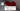# Magical usage of Bitwise operators - Get optimized solutions for many arithmatic problems

October 18, 2019## Introduction

I will list some of the interesting usage of bitwise operators, which looks complex on first look. But, if you understand them. They are so magically wonderful that they appears to be the most optimized solution of problem.

## Some Basics for Bitwise operators

1. Basic operators are:
``````& for AND
| for OR
^ for XOR
>> for right shift
<< for left shift
~ for Negate``````
1. Left or right shift does not change the signed-bit of a number
2. Right shift a number is equivalent to divide a number by 2
3. Left shift a number is equivalent to multiply a number by 2

## Solutions

### 1. Get LSB (Least Significant Digit)

Simply do an & operation with 1 and number.

``````num & 1
# it will simply return either 0 or 1``````

### 2. Counting the number of 1s in a number (Binary)

You need to get the LSB (least significant bit), and check of it is equal to 1.

Code to count number of 1s

``````public int count1s(int num) {
int count = 0;
while (num > 0) {
//or, you can do if (num & 1 != 0)
count += num & 1;
num = num >> 1;
}
return count;
}``````

### 3. Counting number of 1s in optimized way

Above method will run as many times as number of bits in the number. We could simply jump to 1s in the number. How?

Lets see power of masking. We have to target just the 1-bit. We can reset the 1s on LSB side one by one.

``````# example: binary representation: 1010
# we want to reset it to 1000

num & (num - 1)
# i.e. 1010 & 1000 = 1000``````

So, how do we count number of 1s

``````public int count1s(int num) {
int count = 0;
while (num > 0) {
//or, you can do if (num & 1 != 0)
count += num & 1;
num = num & (num - 1);
}
return count;
}``````

### 4. Swap Bits

Given a number, swap ith and jth bit

``````0 1 1 0 0 1 1 0

# swap 2nd and 5th bits (from right)``````

Note: If the bits are different, only then we have to swap them. Else, no need. So, we need to first get those bits. And check for equality. If they are not same, only then swap them. And, what do we mean by swap. We just need to flip their value. To swap, we need to create a mask.

``````# num
i = 2;
j = 5;

if ((num >> i & 1) != (num >> j & 1)) {
# mask for ith, and jth bit
# (1 << i) | (1 << j)

# XOR it with num
num = num ^ ((1 << i) | (1 << j))
}
# else, no need to swap.``````

We optimized above code not to unnecessary swap bits when they are same. We just set ith and jth bit on our mask as 1. And, we will need to XOR it with the number.

``````0 1 1 0 0 1 1 0
-     -
# - denote positions we want to swap